Foxtable(狐表)用户栏目专家坐堂 → [求助]专业报表

共有524人关注过本帖树形打印复制链接

# 主题：[求助]专业报表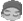hanzhang98
1楼 | 信息 | 搜索 | 邮箱 | 主页 | UC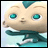加好友发短信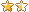[求助]专业报表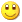Post By：2019/8/16 16:26:00 [只看该作者]

 老师好。想将下面 “代码一” 的帮助文档中 “控制每页打印行数” 的代码，与下面 “代码二” 的专业报表代码结合在一起，以实现控制每页打印行数功能，请老师帮助。谢谢。代码一：（帮助文档中 “控制每页打印行数” 的代码）Dim prs As Integer = 20 '每页20行For p As Integer = 0 To math.Ceiling(tb.Rows.Count / prs) - 1     Dim rt As New prt.RenderTable    rt.Style.Gridlines.All = New prt.Linedef(Color.Gray)    rt.CellStyle.Spacing.All = 0.5    For c As Integer = 0 To tb.Cols.Count - 1        rt.Cells(0,c).Text = tb.Cols(c).Name        For r As Integer = p * prs To math.min(tb.Rows.Count - 1,( p + 1) * prs - 1)            rt.Cells(r - p * prs + 1, c).Text = tb.rows(r)(c)        Next    Next    If p < math.Ceiling(tb.Rows.Count / prs) - 1        rt.BreakAfter = prt.BreakEnum.Page    End If    doc.Body.Children.Add(rt)Next代码二：（专业报表代码）Dim Doc As New PrintDocDim rt As New prt.RenderTable() Dim CurRow As Row = Tables("员工表").Current Dim tb As Table = Tables("员工表")Dim ColNames As String() = New String(){"工号", "姓名", "性别","民族","籍贯","身份证号","出生日期","年龄","户籍地","现住址","联系电话","政治面貌","婚姻状况","学历","资格职称"}'页面设置Doc.PageSetting.PaperKind = 8Doc.PageSetting.Landscape = True Doc.PageSetting.LeftMargin = 14 Doc.PageSetting.RightMargin = 12 Doc.PageSetting.TopMargin = 24 Doc.PageSetting.BottomMargin = 12 '设置主标题rt.Cells(0,0).text = "员工基本信息一览表" rt.Cells(0,0).SpanCols = 15 rt.Cells(0,0).Style.TextAlignHorz = prt.AlignHorzEnum.Center rt.Cells(0,0).Style.Font = New Font("宋体", 20, FontStyle.Bold) rt.Rows(0).Style.Borders.All = New prt.LineDef("0mm", Color.white) rt.Rows(0).Height = 12 rt.Cells(1,0).text = "  单位:" + CurRow("单位名称") rt.Cells(1,0).SpanCols = 15 rt.Cells(1,0).Style.TextAlignHorz = prt.AlignHorzEnum.Left rt.Rows(1).Style.Borders.All = New prt.LineDef("0mm", Color.white) rt.Rows(1).Style.Borders.Bottom = New prt.Linedef  rt.Rows(1).Height = 5 '设置列标题rt.Cells(2,0).SpanRows = 2 '第1行第1个单元格向下合并2行(显示工号)rt.Cells(2,1).SpanCols = 10  '第1行第2列向右合并10个单元格rt.Cells(2,11).SpanRows = 2 '第1行第12个单元格向下合并2行(显示政治面貌)rt.Cells(2,12).SpanRows = 2 '第1行第13个单元格向下合并2行(显示婚姻状况)rt.Cells(2,13).SpanRows = 2 '第1行第14个单元格向下合并2行(显示学历)rt.Cells(2,14).SpanRows = 2 '第1行第15个单元格向下合并2行(显示资格职称)rt.Cells(2,1).Text = "基本信息"  '第一行第一个单元格的内容rt.Cells(2,0).Text= "工号"rt.Cells(3,1).Text = "姓名"rt.Cells(3,2).Text = "性别"rt.Cells(3,3).Text= "民族"rt.Cells(3,4).Text = "籍贯"rt.Cells(3,5).Text = "身份证号"rt.Cells(3,6).Text= "出生日期"rt.Cells(3,7).Text = "年龄"rt.Cells(3,8).Text = "户籍地"rt.Cells(3,9).Text= "现住址"rt.Cells(3,10).Text = "联系电话"rt.Cells(2,11).Text = "政治面貌"rt.Cells(2,12).Text = "婚姻状况"rt.Cells(2,13).Text = "学历"rt.Cells(2,14).Text = "资格职称"'设置每页显示表头rt.RowGroups(2,2).Style.BackColor = Color.LightGray '第1-2行的颜色设为灰色rt.RowGroups(2,2).Style.TextAlignHorz = prt.AlignHorzEnum.Center '第3-4行的文本水平居中rt.RowGroups(2,2).Style.TextAlignVert = prt.AlignVertEnum.Center '第3-4行的文本垂直居中rt.RowGroups(0,4).Header = prt.TableHeaderEnum.All  '前4行作为表头'设置列宽/页宽rt.Width = "Auto" '表格宽度为自动,也就是等于各列设置宽度之和rt.SplitHorzBehavior = prt.SplitBehaviorEnum.SplitIfNeeded '表格宽度超出页宽时,可以水平换页'设置表格字体rt.Style.Font = tb.Font '字体为表格字体rt.Style.TextAlignVert = prt.AlignVertEnum.Center'填入内容For c As Integer = 0 To ColNames.Length - 1 '逐列设置和填入内容    'rt.Cells(0,c).Text = ColNames(c) '列名作为标题    'rt.Cells(0,c).Style.TextAlignHorz = prt.AlignHorzEnum.Center '标题内容水平居中    rt.Cols(c).Width = tb.Cols(ColNames(c)).PrintWidth '列宽等于实际列宽    If tb.Cols(ColNames(c)).IsNumeric OrElse tb.Cols(ColNames(c)).IsDate Then '如果是数值或日期列        rt.Cols(c).Style.TextAlignHorz = prt.AlignHorzEnum.Right '数据水平靠右    End If    For r As Integer = 0 To tb.Rows.Count -1 '开始填入该列内容,从第一行到最后一行        rt.Cells(r + 4, c).Text = tb.Rows(r)(ColNames(c)) '从多层表头以下开始填充第一行(多层表头共4行)    NextNextrt.Style.Gridlines.All = New prt.Linedef(Color.Gray) '灰色网格线rt.CellStyle.Spacing.All = 0.5 '单元格内距设为0.5毫米Doc.Body.Children.Add(rt) '将表格加入到报表doc.Preview() [此贴子已经被作者于2019/8/29 9:31:27编辑过]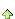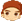2楼 | 信息 | 搜索 | 邮箱 | 主页 | UC加好友发短信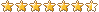Post By：2019/8/16 16:45:00 [只看该作者]

 Dim Doc As New PrintDocDim CurRow As Row = Tables("员工表").CurrentDim tb As Table = Tables("员工表")Dim ColNames As String() = New String(){"工号", "姓名", "性别","民族","籍贯","身份证号","出生日期","年龄","户籍地","现住址","联系电话","政治面貌","婚姻状况","学历","资格职称"}'页面设置Doc.PageSetting.PaperKind = 8Doc.PageSetting.Landscape = TrueDoc.PageSetting.LeftMargin = 14Doc.PageSetting.RightMargin = 12Doc.PageSetting.TopMargin = 24Doc.PageSetting.BottomMargin = 12Dim cnt As Integer = tb.Rows.CountDim prs As Integer = 20 '每页20行For p As Integer = 0 To math.Ceiling(cnt / prs) - 1    Dim rt As New prt.RenderTable()        '设置主标题    rt.Cells(0,0).text = "员工基本信息一览表"    rt.Cells(0,0).SpanCols = 15    rt.Cells(0,0).Style.TextAlignHorz = prt.AlignHorzEnum.Center    rt.Cells(0,0).Style.Font = New Font("宋体", 20, FontStyle.Bold)    rt.Rows(0).Style.Borders.All = New prt.LineDef("0mm", Color.white)    rt.Rows(0).Height = 12        rt.Cells(1,0).text = "  单位:" + CurRow("单位名称")    rt.Cells(1,0).SpanCols = 15    rt.Cells(1,0).Style.TextAlignHorz = prt.AlignHorzEnum.Left    rt.Rows(1).Style.Borders.All = New prt.LineDef("0mm", Color.white)    rt.Rows(1).Style.Borders.Bottom = New prt.Linedef    rt.Rows(1).Height = 5        '设置列标题    rt.Cells(2,0).SpanRows = 2 '第1行第1个单元格向下合并2行(显示工号)    rt.Cells(2,1).SpanCols = 10  '第1行第2列向右合并10个单元格    rt.Cells(2,11).SpanRows = 2 '第1行第12个单元格向下合并2行(显示政治面貌)    rt.Cells(2,12).SpanRows = 2 '第1行第13个单元格向下合并2行(显示婚姻状况)    rt.Cells(2,13).SpanRows = 2 '第1行第14个单元格向下合并2行(显示学历)    rt.Cells(2,14).SpanRows = 2 '第1行第15个单元格向下合并2行(显示资格职称)    rt.Cells(2,1).Text = "基本信息"  '第一行第一个单元格的内容    rt.Cells(2,0).Text= "工号"    rt.Cells(3,1).Text = "姓名"    rt.Cells(3,2).Text = "性别"    rt.Cells(3,3).Text= "民族"    rt.Cells(3,4).Text = "籍贯"    rt.Cells(3,5).Text = "身份证号"    rt.Cells(3,6).Text= "出生日期"    rt.Cells(3,7).Text = "年龄"    rt.Cells(3,8).Text = "户籍地"    rt.Cells(3,9).Text= "现住址"    rt.Cells(3,10).Text = "联系电话"    rt.Cells(2,11).Text = "政治面貌"    rt.Cells(2,12).Text = "婚姻状况"    rt.Cells(2,13).Text = "学历"    rt.Cells(2,14).Text = "资格职称"        '设置每页显示表头    rt.RowGroups(2,2).Style.BackColor = Color.LightGray '第1-2行的颜色设为灰色    rt.RowGroups(2,2).Style.TextAlignHorz = prt.AlignHorzEnum.Center '第3-4行的文本水平居中    rt.RowGroups(2,2).Style.TextAlignVert = prt.AlignVertEnum.Center '第3-4行的文本垂直居中    rt.RowGroups(0,4).Header = prt.TableHeaderEnum.All  '前4行作为表头        '设置列宽/页宽    rt.Width = "Auto" '表格宽度为自动,也就是等于各列设置宽度之和    rt.SplitHorzBehavior = prt.SplitBehaviorEnum.SplitIfNeeded '表格宽度超出页宽时,可以水平换页        '设置表格字体    rt.Style.Font = tb.Font '字体为表格字体    rt.Style.TextAlignVert = prt.AlignVertEnum.Center        '填入内容    For c As Integer = 0 To ColNames.Length - 1 '逐列设置和填入内容        'rt.Cells(0,c).Text = ColNames(c) '列名作为标题        'rt.Cells(0,c).Style.TextAlignHorz = prt.AlignHorzEnum.Center '标题内容水平居中        rt.Cols(c).Width = tb.Cols(ColNames(c)).PrintWidth '列宽等于实际列宽        If tb.Cols(ColNames(c)).IsNumeric OrElse tb.Cols(ColNames(c)).IsDate Then '如果是数值或日期列            rt.Cols(c).Style.TextAlignHorz = prt.AlignHorzEnum.Right '数据水平靠右        End If        For r As Integer = p * prs To math.min(cnt - 1,( p + 1) * prs - 1) '开始填入该列内容,从第一行到最后一行            rt.Cells(r + 4, c).Text = tb.Rows(r)(ColNames(c)) '从多层表头以下开始填充第一行(多层表头共4行)        Next    Next    rt.Style.Gridlines.All = New prt.Linedef(Color.Gray) '灰色网格线    rt.CellStyle.Spacing.All = 0.5 '单元格内距设为0.5毫米    If p < math.Ceiling(cnt / prs) - 1        rt.BreakAfter = prt.BreakEnum.Page    End If    doc.Body.Children.Add(rt)Nextdoc.Preview()hanzhang98
3楼 | 信息 | 搜索 | 邮箱 | 主页 | UC加好友发短信Post By：2019/8/16 17:16:00 [只看该作者]

 谢谢老师。hanzhang98
4楼 | 信息 | 搜索 | 邮箱 | 主页 | UC加好友发短信Post By：2019/8/19 9:08:00 [只看该作者]

 老师好。专业报表――分页小计和合计代码（黄底色部分），小计和合计出的数值都不正确，“本页小计”不是本页的小计、“总计”不是全部的合计，请老师帮助修改。先谢谢。代码如下：Dim Doc As New PrintDocDim CurRow As Row = Tables("工资表").CurrentDim tb As Table = Tables("工资表")Dim ColNames As String() = New String(){"工号", "姓名", "性别","工种或职务","岗序","岗位工资","日资","出勤天","出勤工资","加班工资","节假工资","职称金额","奖金","应发工资"} '指定要打印的列Dim cnt As Integer = tb.Rows.Count '计算总行数Dim prs As Integer = 25 '设置每页25行Dim sum1 As Integer = 0 '岗位工资小计Dim sum2 As Double = 0 '出勤工资小计Dim tsum1 As Integer = 0 '岗位工资合计Dim tsum2 As Double = 0 '出勤工资合计 For p As Integer = 0 To math.Ceiling(cnt / prs) - 1     Dim rt As New prt.RenderTable()     rt.Width = "Auto"  ......            '设置每页显示表头 ......        '设置表格字体 ......        '填入内容    sum1 = 0    sum2 = 0    For c As Integer = 0 To ColNames.Length - 1         If tb.Cols(ColNames(c)).IsNumeric Then             rt.Cols(c).Style.TextAlignHorz = prt.AlignHorzEnum.Right             rt.Style.Font = New Font("Arial Narrow", 7)        End If        For r As Integer = p * prs To math.min(cnt - 1,( p + 1) * prs - 1)             rt.Cells(r+4, c).Text = tb.Rows(r)(ColNames(c))             sum1 =sum1 + tb.Rows(r)("岗位工资")             sum2 =sum2 + tb.Rows(r)("出勤工资")    rt.Style.Gridlines.All = New prt.Linedef(Color.Gray) '灰色网格线    rt.CellStyle.Spacing.All = 0.5 '单元格内距设为0.5毫米    If p < math.Ceiling(cnt / prs) - 1 '如果当前页数小于计算得出的总页数        rt.BreakAfter = prt.BreakEnum.Page '换页    End If   tsum1 = tsum1 + sum1    tsum2 = tsum2 + sum2    rt.Rows.Count = rt.Rows.Count + 1 '增加本页小计行    rt.Cells(rt.Rows.Count -1,0).SpanCols = 6    rt.Rows(rt.Rows.Count -1)(0).Text = "本页小计"     rt.Rows(rt.Rows.Count -1)(6).Text = sum1     rt.Rows(rt.Rows.Count -1)(9).Text = sum2    If p = Math.Ceiling(tb.Rows.Count / prs) - 1        rt.Rows.Count = rt.Rows.Count + 1         rt.Cells(rt.Rows.Count -1,0).SpanCols = 6         rt.Rows(rt.Rows.Count -1)(0).Text = "总计"        rt.Rows(rt.Rows.Count -1)(6).Text = tsum1         rt.Rows(rt.Rows.Count -1)(9).Text = tsum2    Else        rt.BreakAfter = prt.BreakEnum.Page '否则换页    End If    Doc.Body.Children.Add(rt) '将表格加入到报表Nextdoc.Preview() '预览报表 [此贴子已经被作者于2019/8/19 9:13:18编辑过]5楼 | 信息 | 搜索 | 邮箱 | 主页 | UC加好友发短信Post By：2019/8/19 9:34:00 [只看该作者]

 '填入内容    For c As Integer = 0 To ColNames.Length - 1         If tb.Cols(ColNames(c)).IsNumeric Then             rt.Cols(c).Style.TextAlignHorz = prt.AlignHorzEnum.Right             rt.Style.Font = New Font("Arial Narrow", 7)        End If    sum1 = 0    sum2 = 0        For r As Integer = p * prs To math.min(cnt - 1,( p + 1) * prs - 1)             rt.Cells(r+4, c).Text = tb.Rows(r)(ColNames(c))             sum1 =sum1 + tb.Rows(r)("岗位工资")             sum2 =sum2 + tb.Rows(r)("出勤工资")hanzhang98
6楼 | 信息 | 搜索 | 邮箱 | 主页 | UC加好友发短信Post By：2019/8/19 10:18:00 [只看该作者]

 老师好。专业报表――设置行高代码（黄底色部分）不正确，如附图。请老师帮助修改。先谢谢。代码如下：Dim Doc As New PrintDocDim CurRow As Row = Tables("工资表").CurrentDim tb As Table = Tables("工资表")Dim ColNames As String() = New String(){"工号", "姓名", "性别","工种或职务","岗序","岗位工资","日资","出勤天","出勤工资","加班工资","节假工资","职称金额","奖金","应发工资"} '指定要打印的列Dim cnt As Integer = tb.Rows.Count '计算总行数Dim prs As Integer = 25 '设置每页25行 For p As Integer = 0 To math.Ceiling(cnt / prs) - 1     Dim rt As New prt.RenderTable()     '设置表格样式        rt.Width = 398 '设置表格的宽度为398毫米        rt.Rows(2).Height = 8 '设置第3行(列标题第一行)的高度为10毫米    rt.Rows(3).Height = 8 '设置第4行(列标题第二行)的高度为10毫米    For i As Integer = p * prs To math.min(cnt - 1,( p + 1) * prs - 1)         rt.Rows(i+tb.HeaderRows).Height = 7 '设置其余的行高    Next    rt.Style.FontSize = 7 '设置全表字体大小为7磅    rt.Width = "Auto"  ......            '设置每页显示表头 ......        '设置表格字体 ......        '填入内容    For c As Integer = 0 To ColNames.Length - 1         If tb.Cols(ColNames(c)).IsNumeric Then             rt.Cols(c).Style.TextAlignHorz = prt.AlignHorzEnum.Right             rt.Style.Font = New Font("Arial Narrow", 7)        End If        For r As Integer = p * prs To math.min(cnt - 1,( p + 1) * prs - 1)             rt.Cells(r+4, c).Text = tb.Rows(r)(ColNames(c))          Next    Next    rt.Style.Gridlines.All = New prt.Linedef(Color.Gray) '灰色网格线    rt.CellStyle.Spacing.All = 0.5 '单元格内距设为0.5毫米    If p < math.Ceiling(cnt / prs) - 1         rt.BreakAfter = prt.BreakEnum.Page '换页    End If    Doc.Body.Children.Add(rt) '将表格加入到报表Nextdoc.Preview() '预览报表 [此贴子已经被作者于2019/8/19 17:27:54编辑过]7楼 | 信息 | 搜索 | 邮箱 | 主页 | UC加好友发短信Post By：2019/8/19 10:48:00 [只看该作者]

 '填入内容    For c As Integer = 0 To ColNames.Length - 1         If tb.Cols(ColNames(c)).IsNumeric Then             rt.Cols(c).Style.TextAlignHorz = prt.AlignHorzEnum.Right             rt.Style.Font = New Font("Arial Narrow", 7)        End If        For r As Integer = p * prs To math.min(cnt - 1,( p + 1) * prs - 1)             rt.Cells(r+4, c).Text = tb.Rows(r)(ColNames(c))          Next    NextFor i As Integer = p * prs To math.min(cnt - 1,( p + 1) * prs - 1)         rt.Rows(i+tb.HeaderRows).Height = 7 '设置其余的行高    Nexthanzhang98
8楼 | 信息 | 搜索 | 邮箱 | 主页 | UC加好友发短信Post By：2019/8/19 15:01:00 [只看该作者]

 老师好。按老师7楼代码，还是不行，情况与6楼相同。下面是帮助示例中的代码，请老师帮助：1、将控制行高的代码加入。2、如果是数值列，设置数值显示格式为“("#,##0.00")”。谢谢Dim doc As New PrintDocDim tb As Table = Tables("订单")Dim prs As Integer = 20 Dim sum1 As Integer = 0 Dim sum2 As Double = 0 Dim tsum1 As Integer = 0Dim tsum2 As Double = 0 Dim idx1 As Integer= tb.cols("数量").IndexDim idx2 As Integer= tb.cols("金额").IndexFor p As Integer = 0 To math.Ceiling(tb.Rows.Count / prs) - 1    Dim rt As New prt.RenderTable    rt.Style.Gridlines.All = New prt.Linedef(Color.Gray)    rt.CellStyle.Spacing.All = 0.5    sum1 = 0    sum2 = 0    For c As Integer = 0 To tb.Cols.Count - 1        rt.Cells(0,c).Text = tb.Cols(c).Name    Next    For r As Integer = p * prs To math.min(tb.Rows.Count - 1,( p + 1) * prs - 1)        sum1 =sum1 + tb.rows(r)("数量")         sum2 =sum2 + tb.rows(r)("金额")        For c As Integer = 0 To tb.Cols.Count - 1            rt.Cells(r - p * prs + 1, c).Text = tb.rows(r)(c)        Next    Next    tsum1 = tsum1 + sum1    tsum2 = tsum2 + sum2    rt.Rows.Count = rt.Rows.Count + 1    rt.Rows(rt.Rows.count -1)(0).text = "本页小计"    rt.Rows(rt.Rows.count -1)(idx1).text = sum1    rt.Rows(rt.Rows.count -1)(idx2).text = sum2    If p = math.Ceiling(tb.Rows.Count / prs) - 1         rt.Rows.Count = rt.Rows.Count + 1 '增加总计行        rt.Rows(rt.Rows.count -1)(0).text = "总计"        rt.Rows(rt.Rows.count -1)(3).text = tsum1        rt.Rows(rt.Rows.count -1)(6).text = tsum2    Else        rt.BreakAfter = prt.BreakEnum.Page '否则换页    End If    doc.Body.Children.Add(rt)Nextdoc.Preview()9楼 | 信息 | 搜索 | 邮箱 | 主页 | UC加好友发短信Post By：2019/8/19 16:13:00 [只看该作者]

 Dim doc As New PrintDocDim tb As Table = Tables("订单")Dim prs As Integer = 20Dim sum1 As Integer = 0Dim sum2 As Double = 0Dim tsum1 As Integer = 0Dim tsum2 As Double = 0Dim idx1 As Integer= tb.cols("数量").IndexDim idx2 As Integer= tb.cols("金额").IndexFor p As Integer = 0 To math.Ceiling(tb.Rows.Count / prs) - 1    Dim rt As New prt.RenderTable    rt.Style.Gridlines.All = New prt.Linedef(Color.Gray)    rt.CellStyle.Spacing.All = 0.5    sum1 = 0    sum2 = 0    For c As Integer = 0 To tb.Cols.Count - 1        rt.Cells(0,c).Text = tb.Cols(c).Name    Next    For r As Integer = p * prs To math.min(tb.Rows.Count - 1,( p + 1) * prs - 1)        sum1 =sum1 + tb.rows(r)("数量")        sum2 =sum2 + tb.rows(r)("金额")        For c As Integer = 0 To tb.Cols.Count - 1            If tb.Cols(c).IsNumeric                rt.Cells(r - p * prs + 1, c).Text = format(tb.rows(r)(c),"#,##0.00")            Else                rt.Cells(r - p * prs + 1, c).Text = tb.rows(r)(c)            End If        Next    Next    For r As Integer = p * prs To math.min(tb.Rows.Count - 1,( p + 1) * prs - 1)        rt.Rows(r).Height = 7    Next    tsum1 = tsum1 + sum1    tsum2 = tsum2 + sum2    rt.Rows.Count = rt.Rows.Count + 1    rt.Rows(rt.Rows.count -1)(0).text = "本页小计"    rt.Rows(rt.Rows.count -1)(idx1).text = sum1    rt.Rows(rt.Rows.count -1)(idx2).text = sum2    If p = math.Ceiling(tb.Rows.Count / prs) - 1        rt.Rows.Count = rt.Rows.Count + 1 '增加总计行        rt.Rows(rt.Rows.count -1)(0).text = "总计"        rt.Rows(rt.Rows.count -1)(3).text = tsum1        rt.Rows(rt.Rows.count -1)(6).text = tsum2    Else        rt.BreakAfter = prt.BreakEnum.Page '否则换页    End If    doc.Body.Children.Add(rt)Nextdoc.Preview()hanzhang98
10楼 | 信息 | 搜索 | 邮箱 | 主页 | UC加好友发短信Post By：2019/8/19 17:26:00 [只看该作者]

 老师好。还存在问题请教。Dim tb As Table = Tables("工资表")Dim ColNames As String() = New String(){"工号", "姓名", "性别","工种或职务","岗序","岗位工资","日资","出勤天","出勤工资","加班工资","节假工资","职称金额","奖金","应发工资"} '指定要打印的列        For c As Integer = 0 To tb.Cols.Count - 1            If tb.Cols(c).IsNumeric                rt.Cells(r - p * prs + 1, c).Text = format(tb.rows(r)(c),"#,##0.00")            Else                rt.Cells(r - p * prs + 1, c).Text = tb.rows(r)(c)            End If        Next代码中的“tb” 是将绑定表的所有列填入了表格。请教老师，如何将代码中的 “tb” 修改为指定列 “ColNames”。谢谢。 [此贴子已经被作者于2019/8/19 17:26:37编辑过]总数 18 1 2 下一页